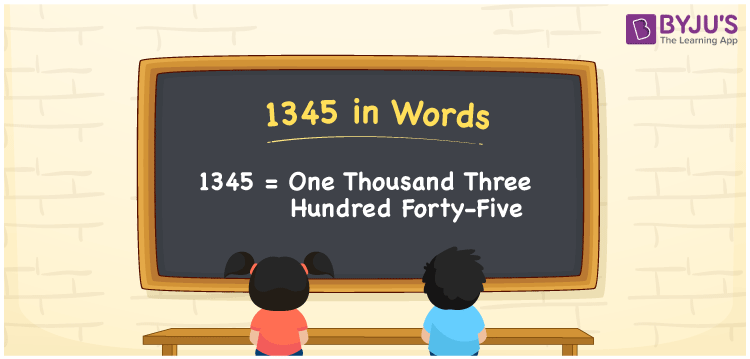# 1345 in words

1345 in words is written as One Thousand Three Hundred and Forty Five. 1345 represents the count or value. The article on Counting Numbers can give you an idea about count or counting. The number 1345 is used in expressions that relate to year, money, distance, length and others. Let us consider an example for 1345. “The Notre Dame Cathedral, Paris was completed in the year One Thousand Three Hundred and Forty Five”.

 1345 in words One Thousand Three Hundred and Forty Five One Thousand Three Hundred and Forty Five in Numbers 1345

## 1345 in English Words## How to Write 1345 in Words?

We can convert 1345 to words using a place value chart. The number 1345 has 4 digits, so let’s make a chart that shows the place value up to 4 digits.

 Thousands Hundreds Tens Ones 1 3 4 5

Thus, we can write the expanded form as:

1 × Thousand + 3 × Hundred + 4 × Ten + 5 × One

= 1 × 1000 + 3 × 100 + 4 × 10 + 5 × 1

= 1345

= One Thousand Three Hundred and Forty Five.

1345 is the natural number that is succeeded by 1344 and preceded by 1346.

1345 in words – One Thousand Three Hundred and Forty Five.

Is 1345 an odd number? – Yes.

Is 1345 an even number? – No.

Is 1345 a perfect square number? – No.

Is 1345 a perfect cube number? – No.

Is 1345 a prime number? – No

Is 1345 a composite number? – Yes

## Solved Example

1. Write the number 1345 in expanded form

Solution: 1 × 1000 + 3 × 100 + 4 × 10 + 5 × 1

We can write 1345 = 1000 + 300 + 40 + 5

= 1 × 1000 + 3 × 100 + 4 × 10 + 5 × 1

## Frequently Asked Questions on 1345 in words

Q1

### How to write 1345 in words?

1345 in words is written as One Thousand Three Hundred and Forty Five.
Q2

### Is 1345 divisible by 5?

Yes. 1345 is divisible by 5.
Q3

### Is 1345 a perfect square number?

No. 1345 is not a perfect square number.